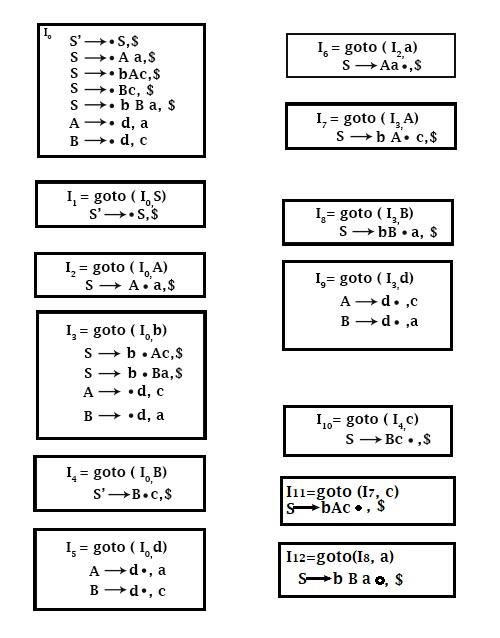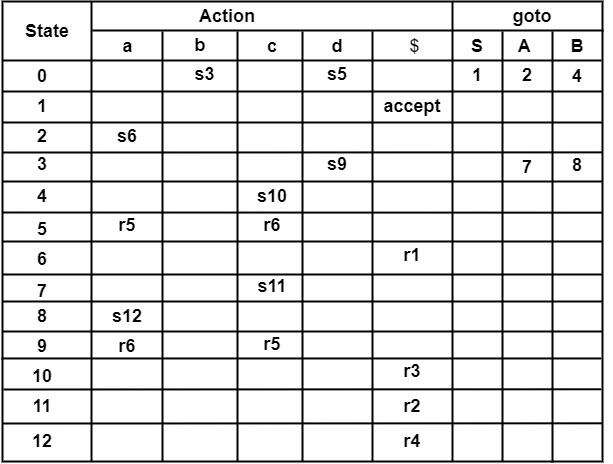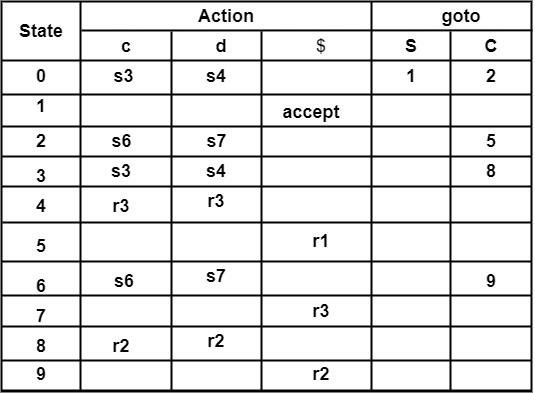# Show that the following grammar is LR (1) S → A a |b A c |B c | b B a A → d B → d

Compiler DesignProgramming LanguagesComputer Programming

## Solution

Step1 − Construct Augment Grammar

(0) S′ → S

(1) S → A a

(2) S → b A c

(3) S → B c

(4) S → b B a

(5) A → d

(6) B → d

Step2 − Find Closure &amp; goto. Construct a set of LR (1) items. Here all the boxes represent new states.LR (1) Parsing TableSo, the LR (1) Parsing Table has no several entries. Grammar is LR (1).

Construction of LR (1) or Canonical LR Parsing Table

Input − An Augmented Grammar G’.

Output − The Canonical LR (1) Parsing Table

Method

Filling the "shift" Entries(s) − Apply Rule (2a) of construction of CLR Parsing Table.

Consider I3 = goto(I0, c)

∴ Action[0, c] = shift 3

∴ Write s3 in front of Row state 0 and column c.

Similarly, consider another entry.

i.e., I7 = goto(I2, d)

∴ Action[2, d] = shift 7

∴ Write s7 in front of Row State 2 and column d. Similarly, other entries of shift are filled onto the Action table.

Filling the "reduce" Entries (r)

Apply rule (2b) of construction of CLR parsing Table. Consider productions of the form A → α ∙ , a

For example, Consider

I4 = goto(I0, d)

C → d ∙, c | d

Here, C → d ∙, c | d is of form A → α ∙ , a. So, set Action [4, c] and Action [4, d] to r3.

Since C → d is production number 3 in the given Question.

∴ Write r3 in front of Row State 4 and column c and d.

Because c, d looks ahead symbols in production C → d ∙ , c | d.

Consider another example

I9 = goto(I9, C)

C → c C ∙, $Since C → c C is production number (2) in the given question. ∴ Write r2 in front of Row State 9 and column$, because $is the lookahead symbol attached to the production. ∴ Action [9,$] = r2

Similarly, fill all entries of reducing into the Parsing Table.

Filling of goto Entries

In goto, only Non-Terminal are seen column-wise,

For example

I8 = goto(I3, C)

∴ Action [3, C] = 8

i.e., write 8 in front of Row state 3 and column C.

Filling of "accept" Entry

Apply Rule (2c) of CLR Parsing Table

Find the state in which production of form S′ → S ∙, $lies ∴ It is state I1 ∴ Write accept in front of Row state 1 &amp; column$.